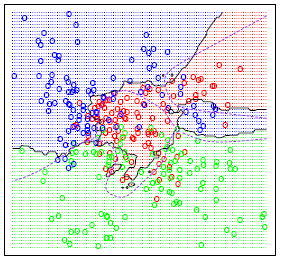# Welcome to STAT 897D!Printer-friendly version

###Welcome to STAT 897D: Applied Data Mining and Statistical Learning!

This course covers methodology, major software tools and applications in data mining. By introducing principal ideas in statistical learning, the course will help students to understand conceptual underpinnings of methods in data mining. It focuses more on usage of existing software packages (mainly in R) than developing the algorithms by the students. Students will be required to work on projects to practice applying existing software. The topics include statistical learning; resampling methods; linear regression; variable selection; regression shrinkage; dimension reduction; non-linear methods; logistic regression, discriminant analysis; nearest-neighbors; decision trees; bagging; boosting; support vector machines; principal components analysis; clustering.

Class announcements and such materials as quizzes, online discussions, and project assignments will be regularly posted on Canvas, so it is recommended that you check Canvas regularly.

### Prerequisites

• STAT 501 (Regression Methods) or a similar course that covers analysis of research data through simple and multiple regression and correlation; polynomial models; indicator variables; step-wise, piece-wise, and logistic regression.
• Basics on probability, expectation, and conditional distributions.
• Matrix algebra and multivariate calculus will be beneficial but is not required.
• The examples in the course use R and students will do weekly R Labs to apply statistical learning methods to real-world data. Extensive guidance in using R will be provided, but previous basic programming skills in R or exposure to a programming language such as MATLAB or Python will be useful.  Introductions to R are available at https://onlinecourses.science.psu.edu/statprogram/node/50 and https://cran.r-project.org/doc/manuals/R-intro.html.

Textbooks:
Required: An Introduction to Statistical Learning, with applications in R (2013), G. James, D. Witten, T. Hastie, R. Tibshirani (Springer).